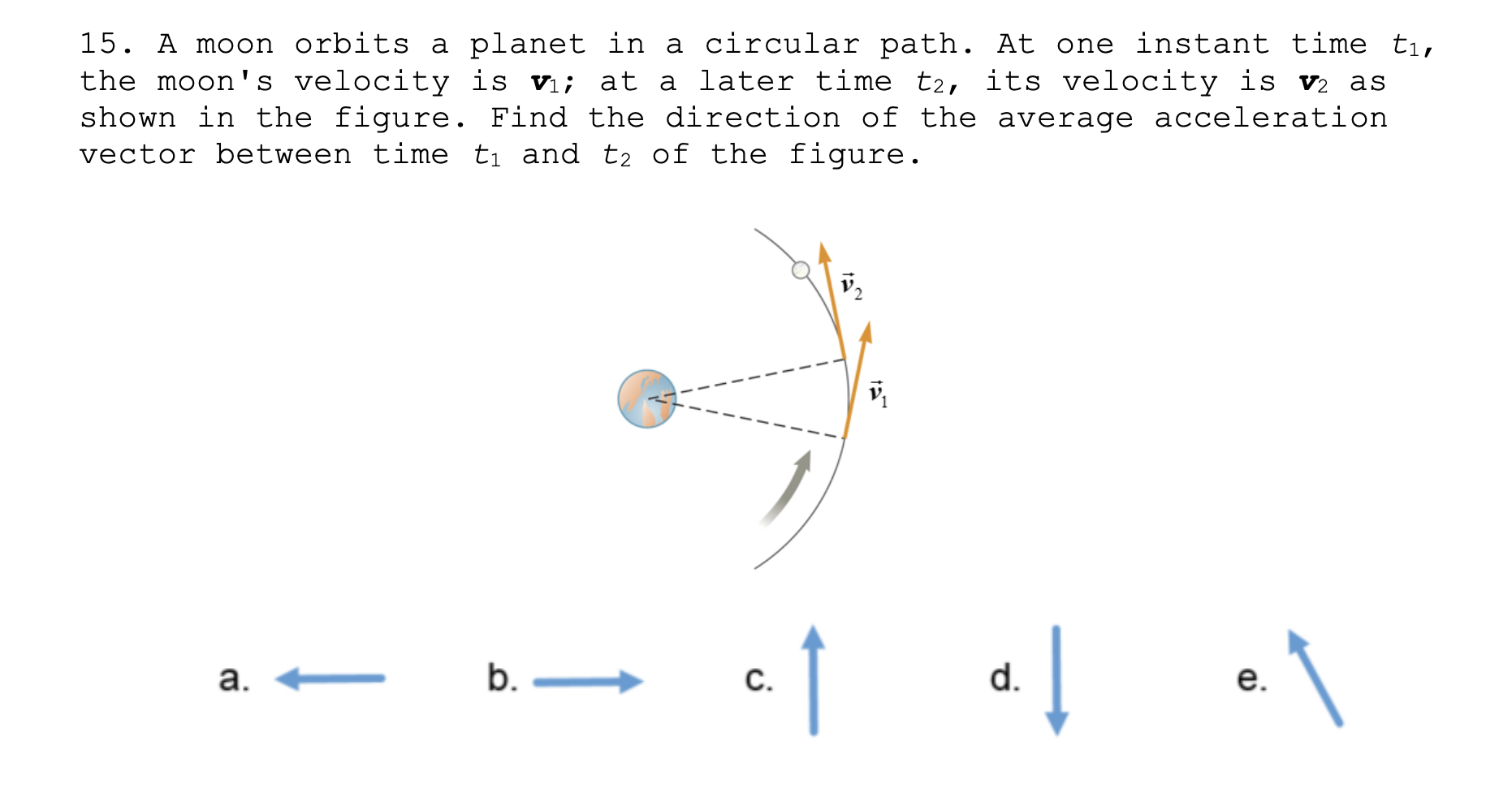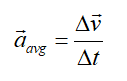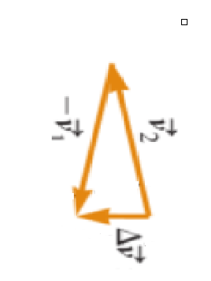# 15. A moon orbits a planet in a circular path. At one instant time ti,the moon's velocity is v; at a later time t2, its velocity is v2 asshown in the figure. Find the direction of the average accelerationvector between time ti and t2 of the figure.b. –d.C.a.e.

Question
1 viewshelp_outlineImage Transcriptionclose15. A moon orbits a planet in a circular path. At one instant time ti, the moon's velocity is v; at a later time t2, its velocity is v2 as shown in the figure. Find the direction of the average acceleration vector between time ti and t2 of the figure. b. – d. C. a. e. fullscreen
check_circle

Step 1

Average acceleration is given asStep 2

To determine the direction of change in velocity, we subtract v2-v1 geometrically.The average velocity is hence towards the center.

...

### Want to see the full answer?

See Solution

#### Want to see this answer and more?

Solutions are written by subject experts who are available 24/7. Questions are typically answered within 1 hour.*

See Solution
*Response times may vary by subject and question.
Tagged in

### Physics